Hurry Up !! Check Out Todays Deal

# Understanding and building simple Neural Network using PyTorch

In this turorial, we’ll go through the basic ideas of PyTorch starting at tensors and computational graphs and finishing at the Variable class and the PyTorch autograd functionality.

## Tensor

Tensor are just multi-dimessional array.Tesnors play a vital role in deep learning libraries for efficient computation.Graphical Processing Unit(GPUs) work effectivly at computing operations between different tensor.In Pytourch there are number of way we can declare tensors:

```import torch a = torch.Tensor(2,3) #create tensor of size(2,3)(or 2x3 matrix) filled with zero b = torch.ones(4,5) #create tensor of size(4,5)(or 2x3 matrix) filled with ones c = torch.rand(5,5) #create tensor of size(5,5)(or 2x3 matrix) filled with random values```

In neural network we calculate errors between predicted ouput and the actual ouput and based on this two we calculate error gradient and do a back-propogation through our computational graph. This mechanisum in PyTorch is called autograd. Autograd allows automatic gradient computation on the tensor when the `.backword()`method is called.

Variable class is the main component autograd system.This class wraps around tensor. The object of this class contains the value of tensor, the gradient of tensor and also contains reference to whatever function created.In PyTorch variable is declared as:

`x = Variable(torch.onses(2,3)+5,requires_grad = True)`

## Creating Neural Network

Here we are going to create a neural network of 4 layer which will consists of 1 input layer,1 ouput layer, and 2 hidden layer. The input consits of 28x28(784) gray scale pixels which are MNIST hand written data set.This input data is passed through 2 hidden layers with ReLU activation function. Finally ouput layer is of 10 nodes corresponding to 10 possibledigit(i.e 0 to 9).At the ouput layer we will be using softmax activation function.

First let import our necessay libraries

```import torch import torch.nn as nn from torch.autograd import Variable import torch.nn.functional as F import torch.optim as optim from torchvision import datasets,transforms from tqdm import tqdm ```

When creating neural network we have to include `nn.Module` class from PyTorch. The `nn.Module` is the base class of all neural network. Inheriting this class allows us to use the functionality of `nn.Module` base class but have the capabilites of overwritting of the base class for model construction/forword pass through our network.

```class Model(nn.Module): def __init__(self): super(Model,self).__init__() self.input_layer = nn.Linear(28*28,200) self.hidde_layer1 = nn.Linear(200,200) self.hidde_layer2 = nn.Linear(200,10)```

In our `__init__()` method we also define our neural net architecture which will contain input layer of nodes 28x28(784), two hidden layer of node 200, and one ouput layer of 10 nodes.

Next lets define how our data will flow in our network. For this we will be using `forword()` in our class. This method will overwrite a dummy method in the base class.and this is needed for definning each network.

``` def forword(self,x): x = F.relu(self.input_layer(x)) x = F.relu(self.hidde_layer1(x)) x = self.hidde_layer1(x) return F.log_softmax(x)```

Now let's write our main function

``` def get_data(batch_size = 200,train = True): data = torch.utils.data.DataLoader( datasets.MNIST('../data', train=train, download=True, transform=transforms.Compose([ transforms.ToTensor(), transforms.Normalize((0.1307,), (0.3081,)) ])), batch_size=batch_size, shuffle=True) return data if __name__ == '__main__': net = Model() net.to(device) optimizer = optim.SGD(net.parameters(),lr = 0.01,momentum = 0.9) criterion = nn.NLLLoss() ## Trainning Model train = get_data() for epoch in tqdm(range(10)): for idx,(x_train,target) in enumerate(train): x_train,target = Variable(x_train).to(device),Variable(target).to(device) x_train = x_train.view(-1,28*28) optimizer.zero_grad() net_out = net(x_train) loss = criterion(net_out,target) loss.backward() optimizer.step() if idx % 10 == 0: print(f"Train Loss {loss.data}") ## Testing our model test = get_data(train = False) test_loss = 0 correct = 0 with torch.no_grad(): for epoch in tqdm(range(10)): for idx,(x_test,target) in enumerate(test): x_test,target = Variable(x_test).to(device),Variable(target).to(device) x_test = x_test.view(-1,28*28) optimizer.zero_grad() net_out = net(x_test) test_loss += criterion(net_out,target).data pred = net_out.data.max(1) correct += pred.eq(target.data).sum() test_loss /= len(test.dataset) print(f"Average loss: {test_loss}\n correct:{correct}\n Accuracy: {(correct/len(test.dataset)) * 100}%") ```

In the main fucntion first we create instance of our model class. Then we define our loss function, here I have used SGD loss function. Next we set our log criterion which is negative log likelihood.

Next in trainnig part i have extracted data from `train` object which is included in PyTourch utility module.This object supplies batched of input. In the outer loop is the number of epochs while the inner loop runs through entire trainning set. The `view` function operates on PyTorch variable to reshape them.`optimizer.zero_grad()` reset all the gradient in this model. `loss.backward()` runs back-propogation operation from loss Variable and `optimizer.step()` method execute gradient descent step based on gradient calculated during the `.backward()` operation.

Similarly we do this operation on test data but now we dont need to update gradient on our network for this we use `torch.no_grad()` to tell PyTorch not to update weight while back-propgating.

After running for 10 epochs I got the following ouput:

```Average loss: 0.0033838008530437946 correct:97720 Accuracy: 98%```

### Realate Post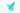#### OpenCV - Contour Detection Using C++

Learn how to use OpenCV to detect contours.We will cover not just the theory, but also complete hands-on coding in C++ for first-hand experience.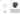React Native Stack Navigator allows your programme to traverse between screens by stacking them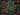#### Javascript String Methods Cheat Sheet 2021

When programming in JavaScript, you often come across scenarios that require string manipulation. For example, when retrieving email , you may need to convert all characters to lowercase or use regular expressions to check if the entered password meets conditions.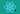#### React 18 is out !

react core team recently released an alpha version of react18. This version pays more attention to user experience and internal architecture changes, including adaptive concurrency functions. It gives you more control over dom rendering events.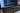#### Nodejs- forEach loop cheatsheet

Node.js forEach executes the supplied function for each element using Node.js forEach. With the help of examples, we will learn how to use the forEach statement in this article.